# Solved Examples: Waves & Sound Waves Notes | Study Mock Test Series for JEE Main & Advanced 2022 - JEE

## JEE: Solved Examples: Waves & Sound Waves Notes | Study Mock Test Series for JEE Main & Advanced 2022 - JEE

The document Solved Examples: Waves & Sound Waves Notes | Study Mock Test Series for JEE Main & Advanced 2022 - JEE is a part of the JEE Course Mock Test Series for JEE Main & Advanced 2022.
All you need of JEE at this link: JEE

Question 1: A sound wave of 42.0-cm wavelength enters the tube shown in the below figure. What must be the smallest radius r such that a minimum will be heard at the detector?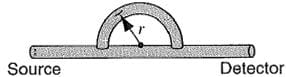Concept:
A minimum sound will be heard at the detector if the sound waves coming from the curve part and the straight part is equal to the half the wavelength of the sound waves.
Solution:
The corresponding figure of the sound waves in the passing through the curve part and straight part is shown below: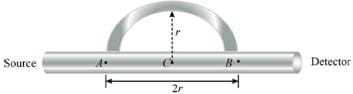Consider the sound waves start from the point A. The sound waves will meet at the point B and interfere and can be detected.
The path length of the sound waves passing through the curve is equal to half the circle having radius r centered at C.
Thus, the path length of the sound waves in the curve part is
L1 = πr
The path length of the sound in the tube ABC is equal to the diameter of the circle having radius r centered at C. So, the path length of the sound waves traveling in it is
L2 = 2r
Now, the path difference of the sound waves at the point C is
Δp = L1- L2
= πr – 2r
= (π – 2)r
For the sound to be heard minimum at the detector, the difference in path length of the sound waves is
Δp = λ/2
Insert Δp = (π – 2)r in the above equation gives
Δp = λ/2
(π – 2)r = λ/2
r = λ/[2(π-2)]
Substitute 42.0 cm  forλ  in the above equation gives
r = λ/[2(π-2)]
= [(42.0 cm) (10-2 m/1 cm)]/2(3.14-2)
= 18.421×10-2 m
Rounding off to three significant figures, the smallest radius of the curve part of the tube to be heard minimum sound at the detector is 18.4×10-2 m.

Question 2: Two stereo loud speakers are separated by a distance of 2.12 m. Assume that the amplitude of the sound from each speaker is approximately the same at the position of a listener, who is 3.75 m directly in front one of the speakers; see below figure. (a) For what frequencies in the audible range (20-20,000 Hz) will there be a minimum signal? (b) For what frequencies is the sound a maximum?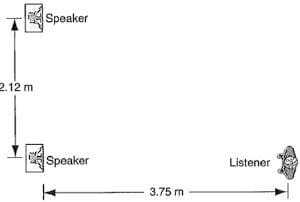Concept:
The signal observed will be minimum when the path difference between the signals from the sources is equal to half integral multiple of the wavelength.
The signal observed will be maximum when the path difference between the signals from the sources is equal to the integral multiple of the wavelength.
Solution:
(a) The corresponding figure for the signals coming from the sources is shown below: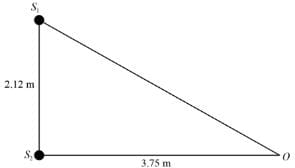From the figures, the two speaker are labeled as Sand S2. The listener is labeled as O.
From the triangle S1 S2O, the path S1O is
S1O = √[(S1 S2)2 + (S2O)2]
= √[(2.12 m)2 + (3.75 m)2]
= 4.3078 m
Now, the path difference between the signal coming from the speakers S1 and S2 is
Δp = S1O - S2O
= 4.31 m – 3.75 m
= 0.56 m
The signal observed by the listener will be minimum when the path difference is equal to the half integral multiple of the wavelength λ.
Δp = (n - ½ ) λ
Here, n = 1,2,3….
The wavelength of the sound wave is
λ = v/f
Here, speed of the sound waves is  and frequency of the sound wave is f.
Insert λ = v/f in the equation Δp = (n - ½ ) λ gives
Δp = (n - ½ ) λ
= (n - ½ ) (v/f)
f = (n - ½ ) (v/Δp)
The speed of the sound waves in air at room temperature is 343 m/s.
Substitute 343 m/s  forv and 0.56 m for Δp in the equation  f = (n - ½ ) (v/Δp) gives
f = (n - ½ ) (v/Δp)
= (n - ½ ) [(343 m/s)/(0.56 m)] (1 Hz.s)
= (n – ½) 612.5 Hz
Rounding off to three significant figures, the frequencies of the speakers should
(n – ½) 613 Hz for the single to be minimum.
(b) The signal observed by the listener will be maximum when the path difference is equal to the integral multiple of the wavelength λ.
Δp = nλ
Here, n = 1,2,3…..
The wavelength of the sound wave is
λ = v/f
Here, speed of the sound waves is v and frequency of the sound wave is f.
Insert λ = v/f  in the equation Δp = nλ gives
Δp = nλ
= n (v/f )
f = n (v/ Δp)
The speed of the sound waves in air at room temperature is 343 m/s.
Substitute 343 m/s for v and 0.56 m for Δp  in the equation f = n (v/ Δp), gives
f = n (v/ Δp)
= n [(343 m/s)/(0.56 m)] (1 Hz.s)
= n (612.5 Hz)
Rounding off to three significant figures, the frequencies of the speakers should
n (613 Hz) for the single to be maximum.

Question 3: S in below figure is a small loudspeaker driven by an audio oscillator and amplifier, adjustable in frequency from 1000 to 2000 Hz only. D is a piece of cylindrical sheet metal pipe 45.7 cm long and opens at both ends. (a) At what frequencies will resonance occur when the frequency emitted by the speaker is varied from 1000 to 2000 Hz? (b) Sketch the displacement nodes for each resonance. Neglect end effects.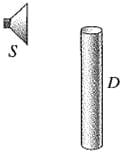Concept:
The given cylindrical sheet metal pipe is equivalent to open tube pipes.
The resonant frequency of the open tube pipe is
f= n (v/2L)
Here, n = 1,2,3…..., speed of the sound in the tube is v and length of the tube is L.
For corresponding frequencies emitted by the small loudspeaker, the tube will resonate.
Solution:
(a) The speed of the sound in air at room temperature is 343 m/s.
Substitute 343 m/s  forv and 45.7 cm for  in the equation f= n (v/2L) gives
fn = n [v/2L]
= n  [ (343 m/s)/2 (45.7 cm) (10-2 m/1 cm)]
= n (375.275/s) (1 Hz.s)
= n (375.275 Hz)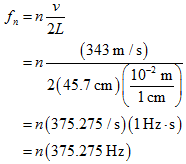For the first harmonic, insert n = 1 in the equation fn = n (375.275 Hz)
gives
fn = n (375.275 Hz)
f1 = (1) (375.275 Hz)
= 375.275 Hz
For the second harmonic, insert n = 2 in the equation fn = n (375.275 Hz)  gives
f2 = (2) (375.275 Hz)
= 750.55 Hz
For the third harmonic, insert n = 3 in the equation f= n (375.275 Hz) gives
f3 = (3) (375.275 Hz)
= 1125.825 Hz
For the fourth harmonic, insert n = 4 in the equation fn = n (375.275 Hz)  gives
f4 = (4) (375.275 Hz)
= 1501.1 Hz
For the fifth harmonic, insert n = 5 in the equation fn = n (375.275 Hz)   gives
f5 = (5) (375.275 Hz)
= 1876.375 Hz
For the sixth harmonic, insert n = 6 in the equation fn = n (375.275 Hz) gives
f6 = (6) (375.275 Hz)
= 2251.65 Hz
It is given that the frequency emitted by the small loudspeaker is varied from 1000 Hz to 2000 Hz. This means the tube will oscillate with frequencies lies between 1000 Hz to 2000 Hz. So, the first, second and sixth harmonics are not resonating with the tube as their frequencies leis beyond the given range. Only third, fourth and fifth harmonic will resonate.
Rounding off to three significant figures, the tube will resonate at frequencies 1130 Hz, 1500 Hz, and 1880 Hz.
(b) For n = 3, the displacement nodes are shown below: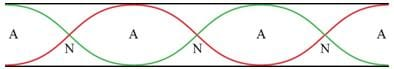For n = 4, the displacement nodes are shown below: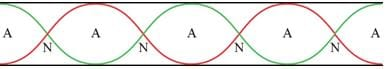For n = 5, the displacement nodes are shown below: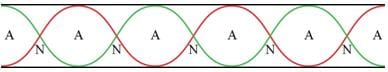The document Solved Examples: Waves & Sound Waves Notes | Study Mock Test Series for JEE Main & Advanced 2022 - JEE is a part of the JEE Course Mock Test Series for JEE Main & Advanced 2022.
All you need of JEE at this link: JEEUse Code STAYHOME200 and get INR 200 additional OFF

## Mock Test Series for JEE Main & Advanced 2022

2 videos|258 docs|160 tests

Track your progress, build streaks, highlight & save important lessons and more!

,

,

,

,

,

,

,

,

,

,

,

,

,

,

,

,

,

,

,

,

,

;